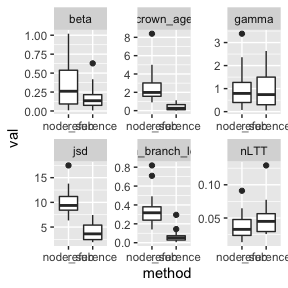NodeSub workflow

Given a tree, we can generate a NodeSubstitution alignment as follows (please note that for this example, we use an extremely short sequence length to reduce simulation time. Such short sequence lengths might not be appropriate for more serious applications.)

seq_length <- 100
sub_rate <- 1 / seq_length

input_tree <- TreeSim::sim.bd.taxa(n = 100,
numbsim = 1,
lambda = 1,
mu = 0.1,
complete = TRUE)[]

rate1 = sub_rate,
rate2 = sub_rate,
l = seq_length,
node_time = 0.3)

We can then proceed to create a Twin alignment (e.g. an alignment with the exact same number of accumulated substitutions, given the same tree, but using a ‘normal’ substitution model)

comp_alignment <- create_equal_alignment(input_tree = geiger::drop.extinct(input_tree),  # can only work on trees without extinct branches
sub_rate = sub_rate,
alignment_result = target_alignment)

Now we have two alignments, one generated using the Node Substitution model, and one using standard strict clock model. For both we can perform phylogenetic inference to get the resulting tree. The aim is to compare the posterior distribution of trees with the true tree that we started with ‘input_tree’, and estimate the error invoked by the node substitution model.

if (beastier::is_beast2_installed()) {

node_posterior <- infer_phylogeny(target_alignmentalignment, "node_posterior", clock_prior = beautier::create_strict_clock_model(clock_rate_param = beautier::create_clock_rate_param(value = sub_rate)), chain_length = 1e5, burnin = 0.1, working_dir = getwd(), sub_rate = sub_rate) reference_posterior <- infer_phylogeny(comp_alignmentalignment,
"reference_posterior",
burnin = 0.1,
clock_prior = beautier::create_strict_clock_model(clock_rate_param = beautier::create_clock_rate_param(value = comp_alignmentadjusted_rate)), chain_length = 1e5, working_dir = getwd, sub_rate = sub_rate) } ## Warning in infer_phylogeny(target_alignmentalignment, "node_posterior", : WARNING! MCMC chain not converged!
## remaining 2
## total_trees 21
## Warning in infer_phylogeny(comp_alignmentalignment, "reference_posterior", : WARNING! MCMC chain not converged! ## remaining 2 ## total_trees 21 Having two posterior distributions of trees, we can compare them based on summary statistics. if (beastier::is_beast2_installed() == TRUE) { node_stats <- calc_sum_stats(node_posteriorall_trees,
input_tree)
ref_stats  <- calc_sum_stats(reference_posterior$all_trees, input_tree) node_stats$differences$method <- "node_sub" ref_stats$differences$method <- "reference" results <- rbind(node_stats$differences,
ref_stats$differences) } ## Warning in calc_sum_stats(node_posterior$all_trees, input_tree): Found extinct lineages, removed these from tree
## Registered S3 method overwritten by 'apTreeshape':
##   method          from
##   is.binary.phylo ape
## Warning in calc_sum_stats(reference_posterior\$all_trees, input_tree): Found extinct lineages, removed these from tree

We can now visualize the differences.

if (beastier::is_beast2_installed()) {
results %>%
tidyr::gather(key = "statistic", value = "val", -method) %>%
dplyr::filter(statistic != "num_tips") %>%
ggplot(aes(x = method , y = val)) +
geom_boxplot() +
facet_wrap(~statistic, scales = "free")
}We find that the beta, gamma and nLTT statistic yield very similar results. However, the JSD, crown age and mean branch length statistics indicate that when using the node substitution model, errors arise, where the crown age of the trees inferred from the node substitution alignment is too high, resulting in a higher mean branch length and a difference in the JSD statistic.